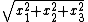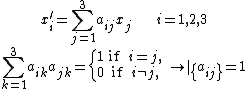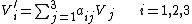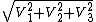# Vector methods

## Vector methods (physics)

Methods that make use of the behavior of physical quantities under coordinate transformations.

From the point of view of physics, the most appropriate definition of a vector in three-dimensional space is a quantity that has three components which transform under rotations of the coordinate system like the coordinates of a point in space. What characterizes rotations is that the distance from the origin—of all points x with cartesian coordinates xi, i = 1, 2, 3—remains unchanged. Specifically, if the rotation takes the xi to new coordinates xi, given by Eqs. (1) [in which det{aij} is
(1)the determinant of the matrix {aij}], then the three quantities Vi, i = 1, 2, 3, form the components of a vector V , if in the new coordinate system the transformed coordinates are given by Eq. (2).
(2)If the coordinate transformation of Eqs. (1) is such that for i = j and aij = 0 for ij, but such that det{aij} = -1 rather than +1 as in Eqs. (1), then it describes a reflection, in which a right-handed coordinate system is replaced by a left-handed one. If, for such a transformation, Eq. (2) also holds, then V is called a polar vector, whereas if the components of V do not change sign, it is called an axial vector or pseudovector. The vector V can also be looked upon as a quantity with a direction, with the magnitudepointing from the origin of the coordinate system to the point in space with the cartesian coordinates (V1, V2, V3).

A quantity that remains invariant under a rotation of the coordinate system is called a scalar. The importance of vectors and scalars in physics derives from the assumed isotropy of the universe, which implies that all general physical laws should have the same form in any two coordinate systems that differ only by a rotation. It is therefore useful to classify physical quantities according to their transformation properties under coordinate rotations. Examples of scalars include the mass of an object, its electric charge, its volume, its surface area, the energy of a system, and its temperature. Other quantities have a direction and thus are vectors, such as the force exerted on a body, its velocity, its acceleration, its angular momentum, and the electric and magnetic fields. Since the sum of two scalars is a scalar, and the sum of two vectors is a vector, it is important in the formulation of physical laws not to mix quantities that have different transformation properties under coordinate rotations. The sum of a vector and a scalar has no simple transformation properties; a law that equated a vector to a scalar would have different forms in different coordinate systems and would thus not be acceptable.

References in periodicals archive ?
The term SVM is typically used to describe classification with support vector methods and support vector regression is used to describe regression with support vector methods.
In order to analyse the characteristics of CRAMM, CORAS, OCTAVE and VECTOR methods, Table 1 presents the suumary of these methods with strenghts and weaknesses.
Table 6 shows the EVAO results based on a comparison of the risk values from the adapted OCTAVE and VECTOR methods .
ii) Two risk assessments methods, namely, the OCTAVE and VECTOR methods were used in combination to achieve better results in risk assessment.
Barkana, "A comparison of the common vector and the discriminative common vector methods for face recognition", in Proc.
Another new non-linear method, the kernel common vector method (KCV) was developed in (18).
proved that the kernel common vector method is a strong nonlinear subspace classifier for various types of databases like Iris data base, wine data base, face data base etc.
LSI has equaled or out-performed standard vector methods and other variants in every case, with improvement of as much as 30%.
Thus, the same terms and term weights were used for both the standard vector and LSI vector methods.
Osten, "Normal vector method for convergence improvement using the RCWA for crossed gratings," Journal of the Optical Society of America A, Vol.
In the vector method, the sets of as many equations of type (1) was created as existed the vectors having solution at [t.
Unfortunately, in vector method, there were assumed that every equation (7) is valid on the entire length of [V.

Site: Follow: Share:
Open / Close